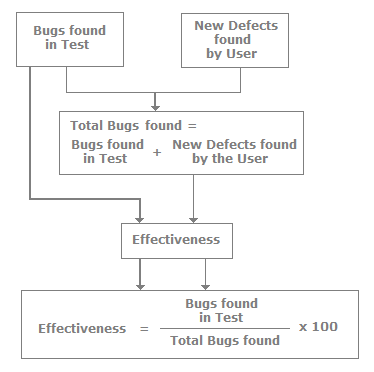1. What is meant by measures and metrics?

Measures are quantitatively unit defined elements, for instance, hours, km, etc. Metrics are basically comprised of more than one measure. For instance, we can have metrics such as km/hr, m/s etc.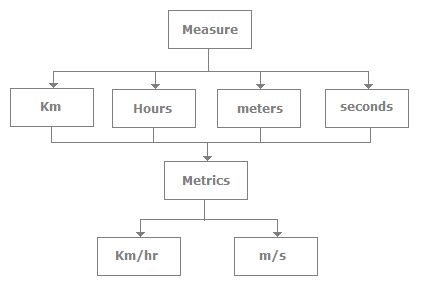2. Can you explain how the number of defects is measured?

The number of defects is one of the measures used to measure test effectiveness. One of the side effects of the number of defects is that all bugs are not equal. So it becomes necessary to weight bugs according to their criticality level. If we are using the number of defects as the metric measurement the following are the issues:

• The number of bugs that originally existed significantly impacts the number of bugs discovered, which in turns gives a wrong measure of the software quality.
• All defects are not equal so defects should be numbered with a criticality level to get the right software quality measure.

3. Can you explain unit and system test DRE?

DRE is also useful to measure the effectiveness of a particular test such as acceptance, unit, or system testing. The following figure shows defect numbers at various software cycle levels. The 1 indicates that defects are input at the phase and2indicates that these many defects were removed from that particular phase. For instance, in the requirement phase 100 defects were present, but 20 defects are removed from the requirement phase due to a code review. So if 20 defects are removed then 80 defects get carried to the new phase (design) and so on.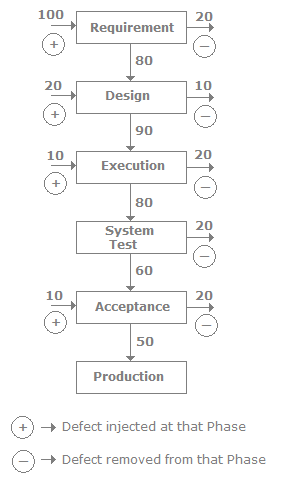4. Can you explain DRE?

DRE (Defect Removal Efficiency) is a powerful metric used to measure test effectiveness. From this metric we come to know how many bugs we found from the set of bugs which we could have found. The following is the formula for calculating DRE. We need two inputs for calculating this metric: the number of bugs found during development and the number of defects detected at the end user.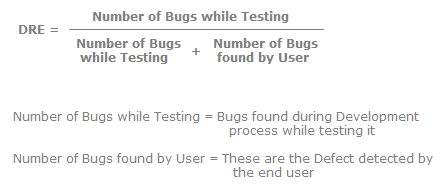But the success of DRE depends on several factors. The following are some of them:

• Severity and distribution of bugs must be taken into account.
• Second, how do we confirm when the customer has found all the bugs. This is normally achieved by looking at the history of the customer.

5. Can you explain defect age and defect spoilage?

Defect age is also called a phase age or phage. One of the most important things to remember in testing is that the later we find a defect the more it costs to fix it. Defect age and defect spoilage metrics work with the same fundamental, i.e., how late you found the defect. So the first thing we need to define is what is the scale of the defect age according to phases. For instance, the following table defines the scale according to phases. So, for instance, requirement defects, if found in the design phase, have a scale of 1, and the same defect, if propagated until the production phase, goes up to a scale of 4.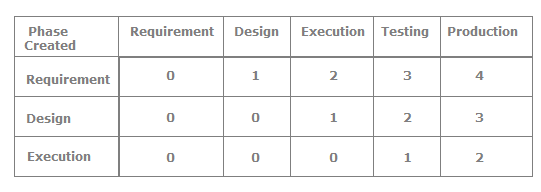Once the scale is decided now we can find the defect spoilage. Defect spoilage is defects from the previous phase multiplied by the scale. For instance, in the following figure we have found 8 defects in the design phase from which 4 defects are propagated from the requirement phase. So we multiply the 4 defects with the scale defined in the previous table, so we get the value of 4. In the same fashion we calculate for all the phases. The following is the spoilage formula.6. Can you explain how the number of production defects is measured?

This is one of the most effective measures. The number of defects found in a production is recorded. The only issue with this measure is it can have latent and masked defects which can give us the wrong value regarding software quality.

7. Can you explain defect seeding?

Defect seeding is a technique that was developed to estimate the number of defects resident in a piece of software. It’s an offline technique and should not be used by everyone. The process is the following: we inject the application with defects and then see if the defect is found or not. So, for instance, if we have injected 100 defects we try to get three values. First how many seeded defects were discovered, how many were not discovered, and how many new defects (unseeded) are discovered. By using defect seeding we can predict the number of defects remaining in the system.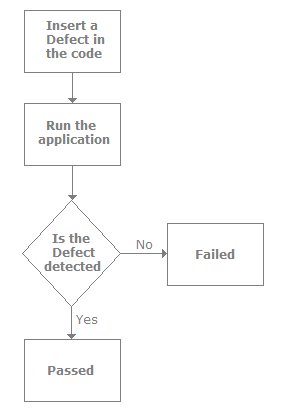8. How do you measure test effectiveness?

Test effectiveness is the measure of the bug-finding ability of our tests. In short, it measures how good the tests were. So effectiveness is the ratio of the measure of bugs found during testing to the total bugs found. Total bugs are the sum of new defects found by the user plus the bugs found in the test. The following figure explains the calculations in a pictorial format.